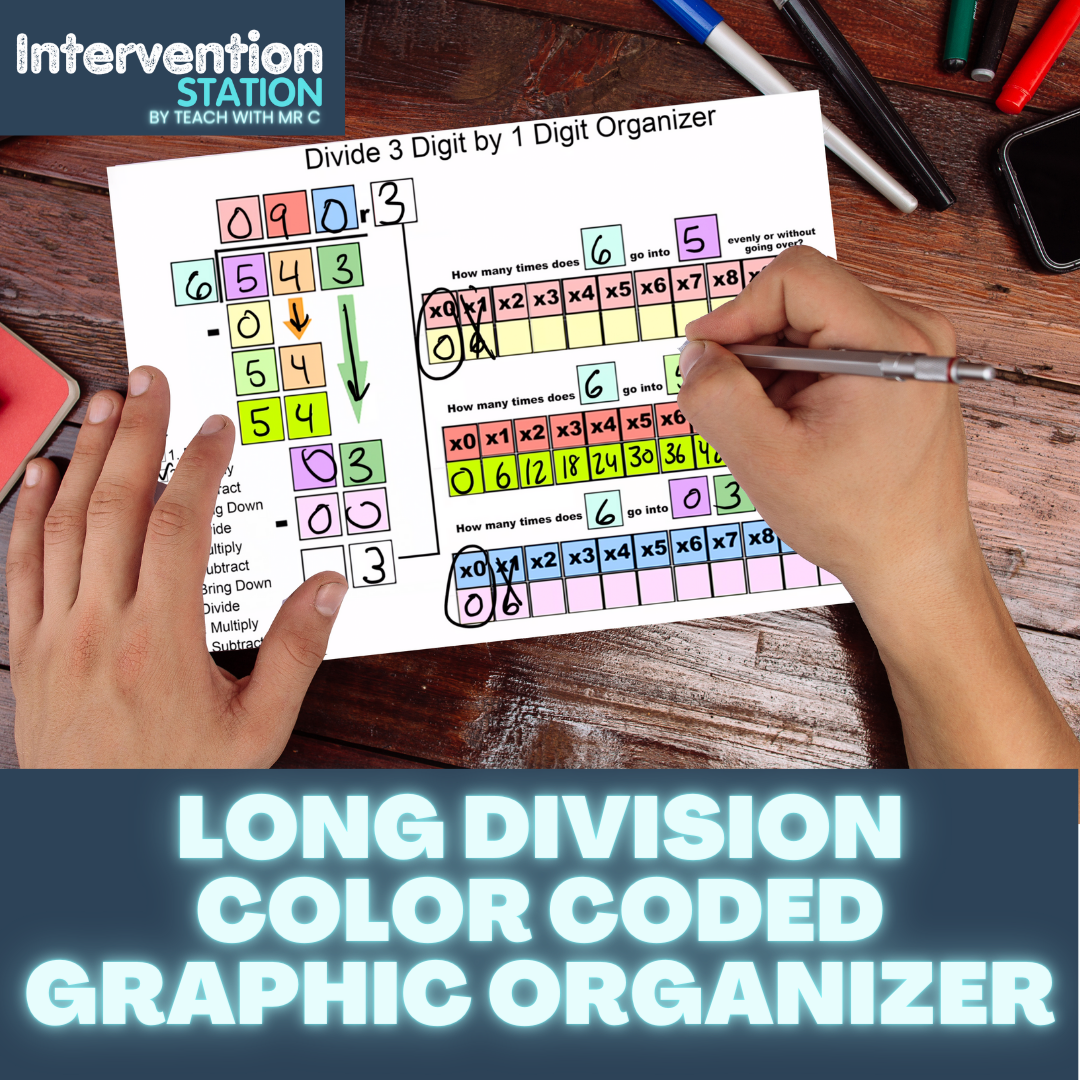#### Color Coded Long Division Graphic Organizers

##### (6 Resources)

These graphic organizers have helped thousands of students master long division. They come in the following format:

• 2 digit by 1
• 3 digit by 1
• 4 digit by 1
• 3 or 4 digit by 2 digits
• Google Sheet Multiple Finder to Help Students Find the Multiples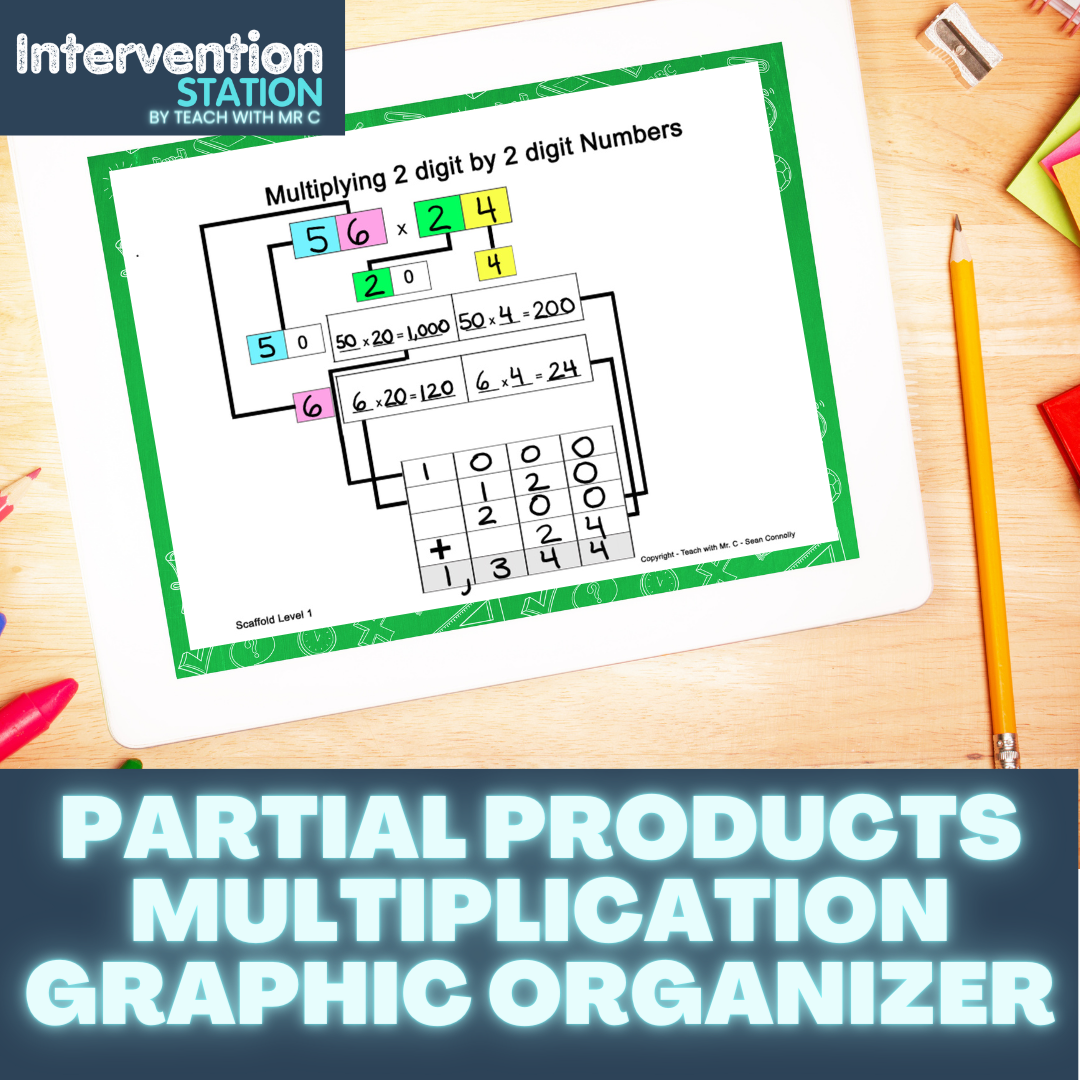#### Multiplication

##### (5 Resources)

Multiplication Color Coded Graphic Organizers

• Partial Products
• 2 digit by 1
• 3 digit by 1
• 4 digit by 1
• 2 digit by 2 digit
• Standard Algorithm Color Coded Graphic Organizers
• 2 digit by 2 digit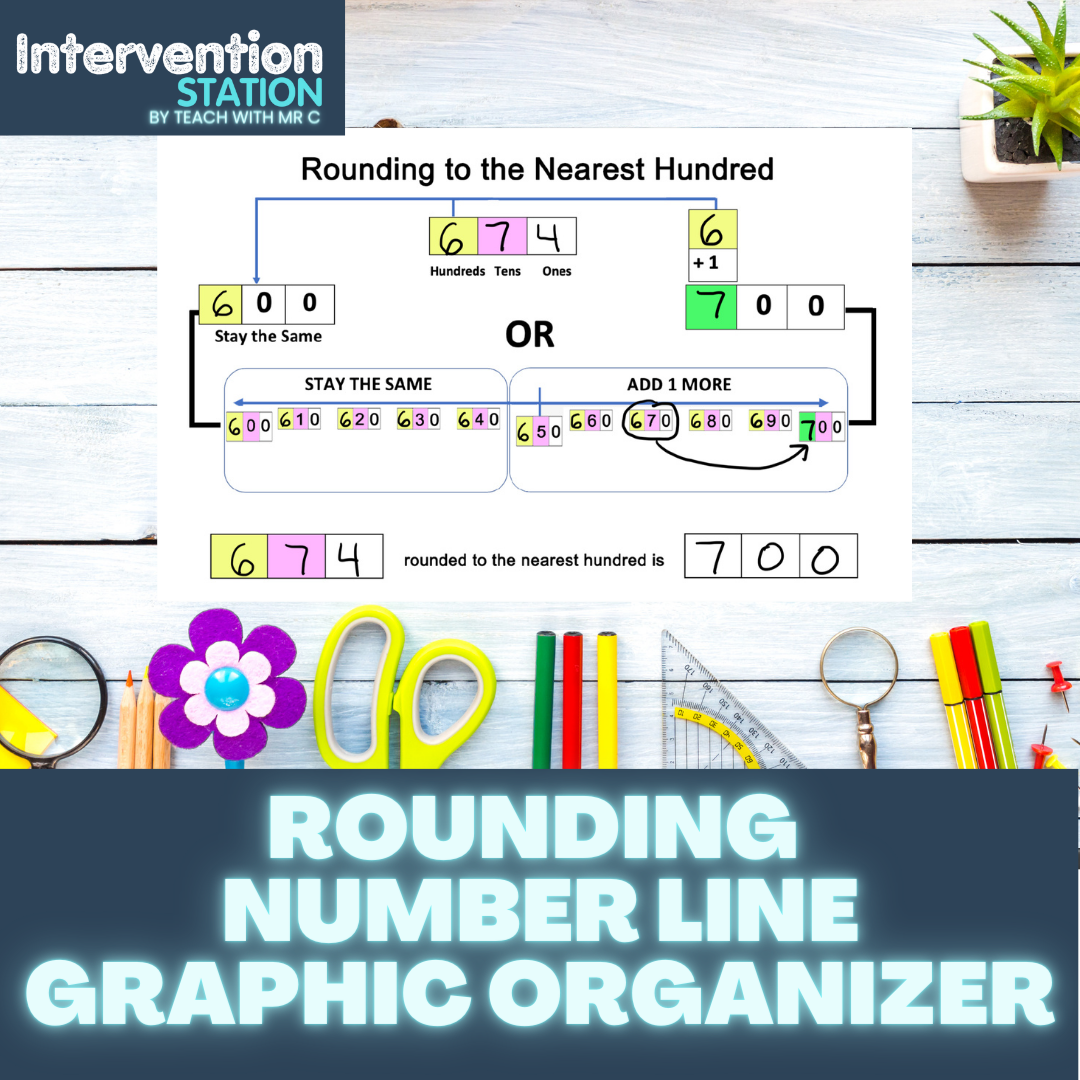#### Rounding

##### (9 Resources)
• Round to the nearest ten
• Round to the nearest ten with a hundred number
• Round to the nearest hundred
• Round to the nearest hundred in a thousand number
• Round to the nearest thousand
• Round to the nearest thousand in a ten thousand number
• Rounding decimals to the nearest whole number
• Rounding decimals to the nearest tenth
• Rounding decimals to the nearest hundredth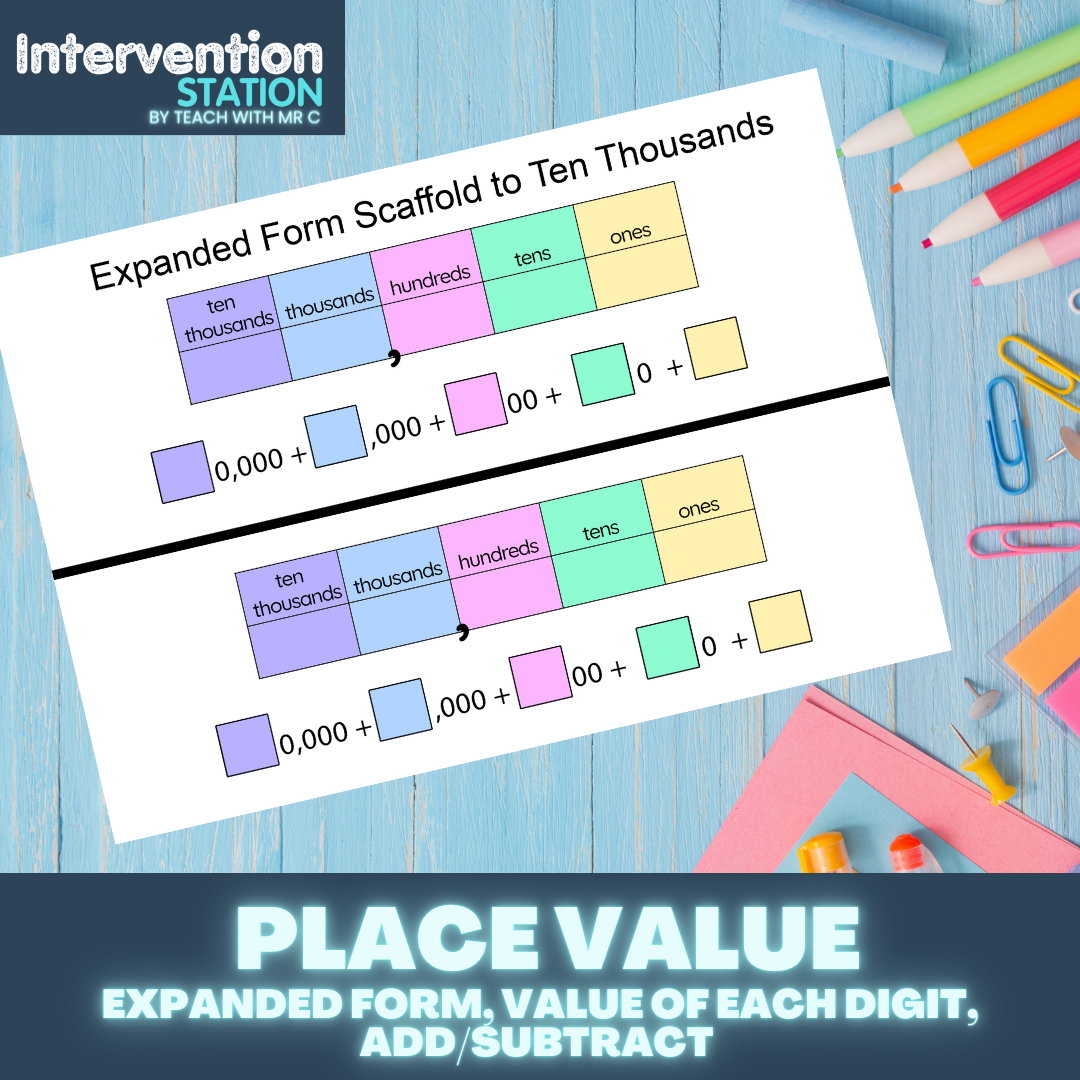#### Place Value

##### (14 resources)
• Blank Place Value Chart Color Coded
• Place Value Dice Game to Practice Expanded, Standard and Word Form
• Reading and Writing Numbers up to millions
• Expanded Form to Millions
• Comparing Numbers up to Millions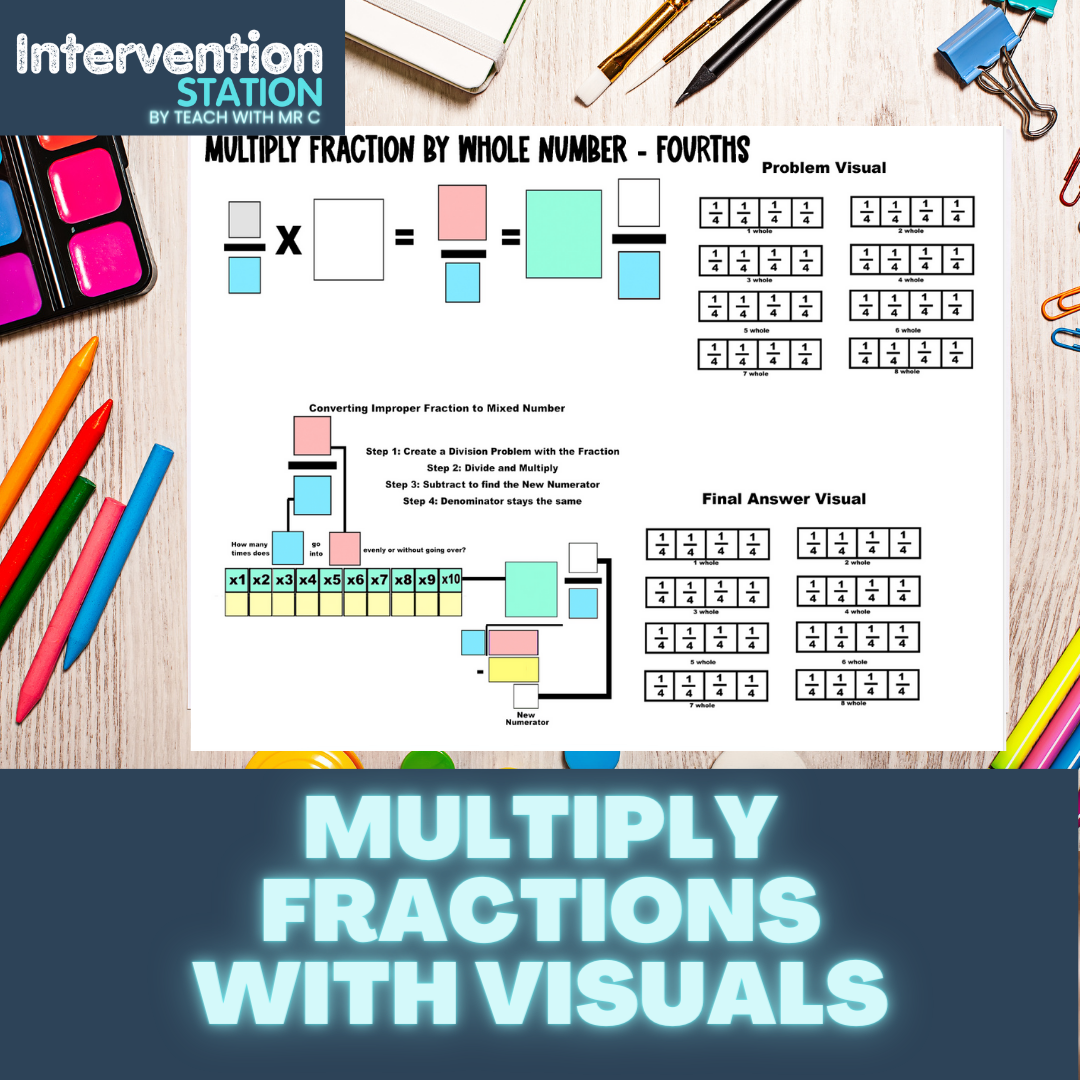#### Fractions

##### (9 Resources)
• Least Common Denominator
• Equivalent Fractions Number Line
• Subtract Fractions with Visual Model
• Subtract Mixed Numbers with Borrowing
• Compare Fractions
• Convert Mixed Numbers to Improper Fractions
• Convert Improper Fractions to Mixed Numbers with Division
• Converting Mixed Numbers with Improper Fractions to Proper Fractions
• Multiply Fraction by Whole Number with Visual Model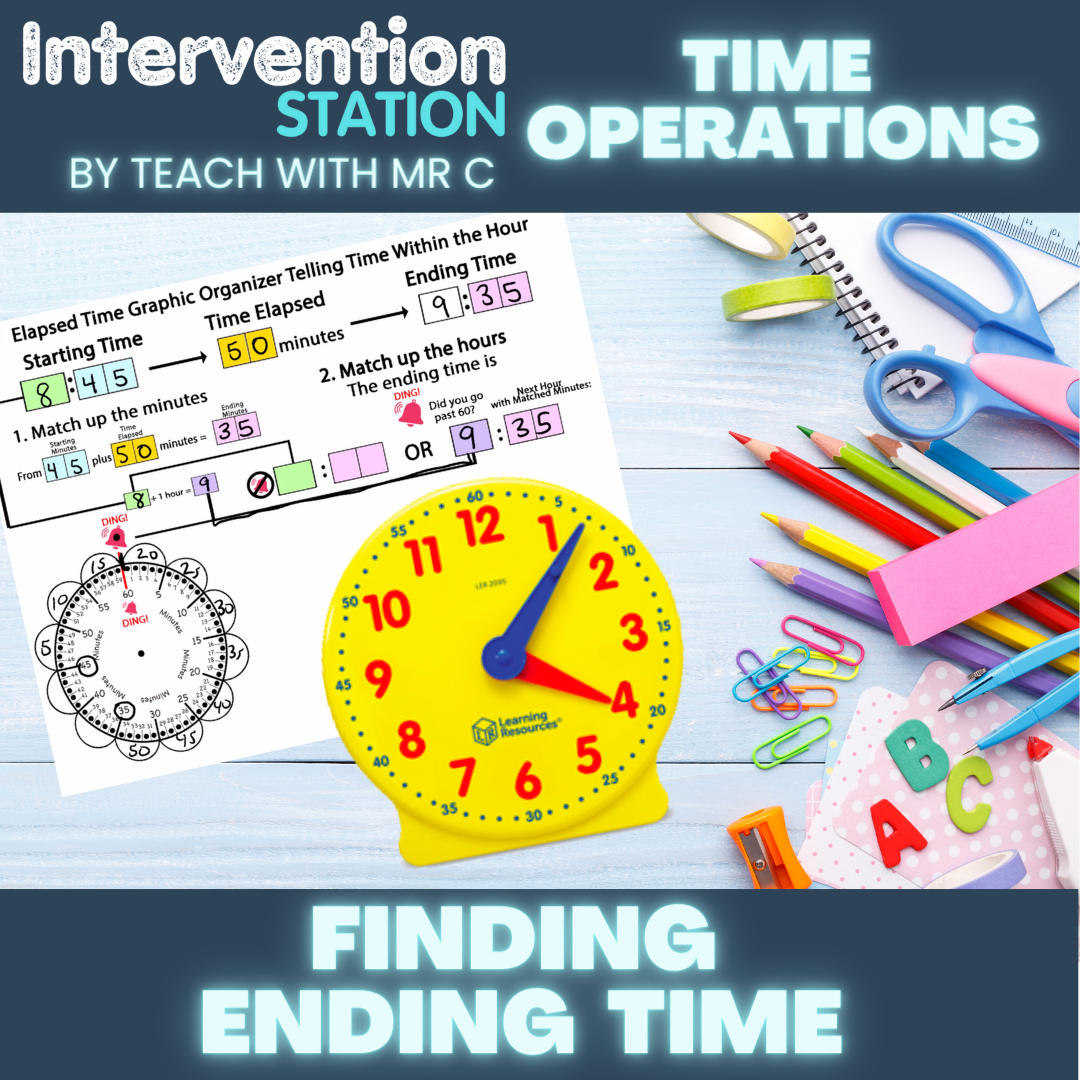#### Time

• Time

• Finding Elapsed Time
• Finding Ending Time
• Finding Elapsed Time within One Hour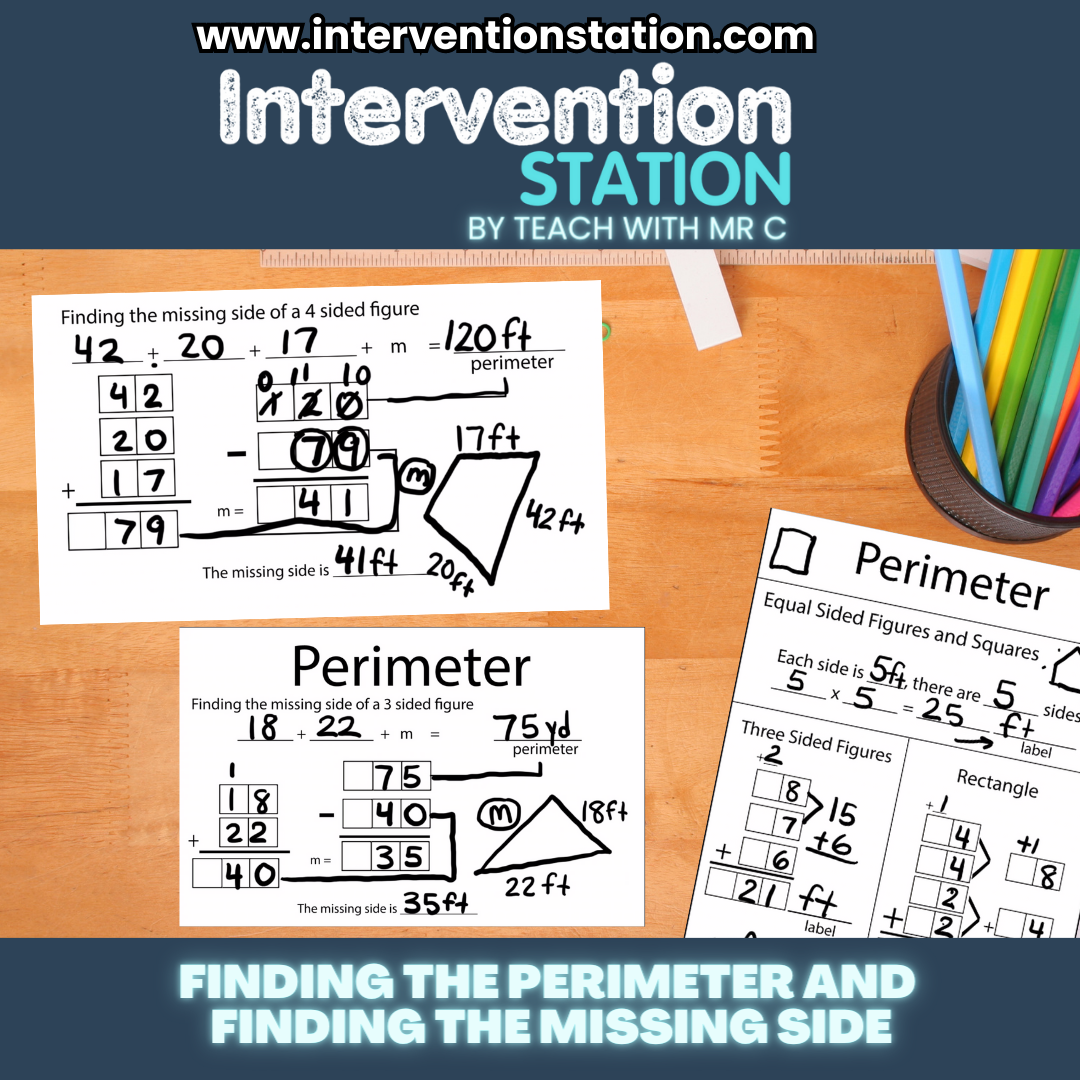#### Perimeter/Geometry

• Find the Perimeter of 3,4,5 and 6 sided figures.
• Find the missing side when given the perimeter for 3,4,5 and 6 sided figures
• Perimeter of Rectangles Formulas and Color Coded Graphic Organizer
• Classify Triangles by Angle Flow Chart
• Classify Triangles by Length Flow Chart
• Classify Angles Flow Chart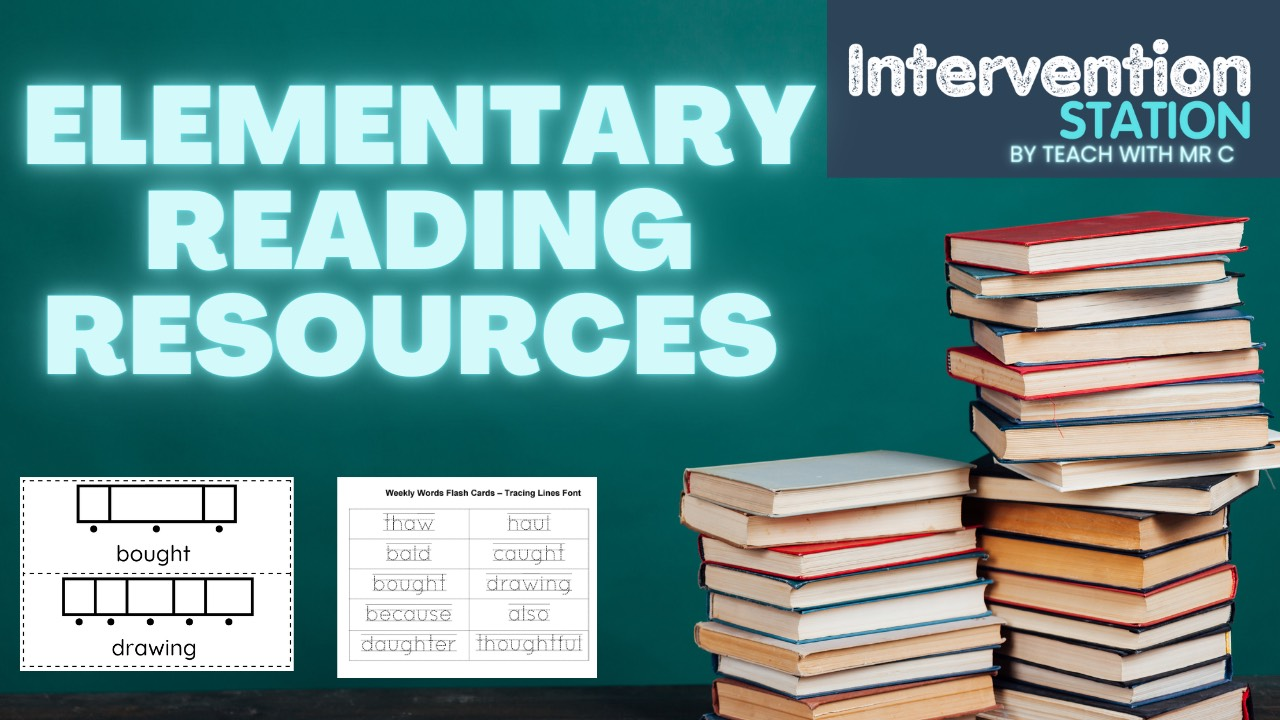#### Auto Generating Phonics and Spelling PDF

• Quickly make word specific sound boxes in seconds
• The sound boxes are for showing students how many letters go in each box
• Create tracing pages in seconds
• Create shape boxes pages in seconds
• Write sentences and create shape boxes for students to fill in

Intervention Station Resources – More Resources Added Each Week!

Each Resource has individual video attached. Please watch this overview of how to get around the site as a member.

Math Resources

Long Division Color Coded Graphic Organizers

• 2 digit by 1
• 3 digit by 1
• 4 digit by 1
• 3 or 4 digit by 2 digits

Multiplication Color Coded Graphic Organizers

• Partial Products
• 2 digit by 1
• 3 digit by 1
• 4 digit by 1
• 2 digit by 2 digit
• Standard Algorithm Color Coded Graphic Organizers
• 2 digit by 2 digit

Rounding on a Number Line Color Coded Graphic Organizers

• Round to the nearest ten
• Round to the nearest ten with a hundred number
• Round to the nearest hundred
• Round to the nearest hundred in a thousand number
• Round to the nearest thousand
• Round to the nearest thousand in a ten thousand number

Fractions Color Coded Graphic Organizers

• Least Common Denominator
• Compare Fractions
• Convert Mixed Numbers to Improper Fractions
• Convert Improper Fractions to Mixed Numbers with Division
• Converting Mixed Numbers with Improper Fractions to Proper Fractions
• Multiply Fraction by Whole Number

Decimals

• Coming Soon – Adding Tenths and Hundredths Graphic Organizer
• Coming Soon – Comparing Decimals Graphic Organizer

Measurement

• Coming Soon – Converting Measurements
• Convert Inches, Yards, Feet and Miles
• Convert Ounces, Pounds and Tons
• Convert Volume – Fluid Ounces, Cups, Pints, Quarts and Gallons
• Convert Metric Length – Centimeters, Millimeters, Meters, Kilometers
• Convert Metric Mass – Milligrams, Grams and Kilograms
• Convert Metric Volume – milliliters, liters

Time

• Coming Soon – Telling Time Visual
• Coming Soon – Elapsed Time Color Coded Graphic Organizer